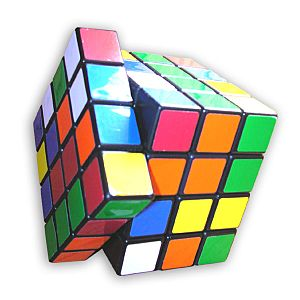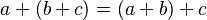# Group (mathematics) facts for kids

Kids Encyclopedia FactsA Rubik's Revenge cube. All the possible positions of the cube, if paired with a suitable operation, would form a group.

In mathematics, a group is a kind of algebraic structure. A group has a set and an operation. The group's operation can combine any two elements of the group's set. This forms a third element.

Instead of "an element of the group's set", mathematicians usually save words. They say "an element of the group".

Mathematicians use capital letters to stand for groups. They often use G, H, or K.

They use lower-case letters to stand for group elements. To save words, they say "a is in G" to mean "a is an element of G".

They write group operations with symbols like • or *, or by writing two elements next to each other. So "ab", "a * b", and "ab" can all mean "the element formed when the group's operation combines a and b".

Not every set and operation make a group. A group's set and operation must obey some special rules. These are called group axioms. This list has each axiom twice, once in words, and once in mathematical symbols.

## Group axioms

• Closure: When a group's operation combines two elements, the element that is formed must also be part of the group.
• For all a, b in G, ab is also in G.
• Identity element: One element of the group is special. It is called the identity element. If the operation combines the identity element and any second element, the answer will be that second element. The identity element is usually written as e. Sometimes the identity element is written as 0 or 1.
• There exists e in G, so that for all a in G, ea = ae = a.
• Associativity: When the operation is used twice to combine three elements, it does not matter in what order they are done, because the answer will be the same.
• For all a, b and c in G, (ab) • c = a • (bc).
• Inverse element: Every element in the group has another element in the group that is called its inverse. When the operation combines any element and its inverse, the answer is the identity element. Sometimes the inverse is indicated by writing either a - in front or by writing -1 superscript, so the inverse of the element a is either -a or a-1.
• For each a in G, there must exist b in G, so that ab = ba = e, where e is G's identity element.

## Some more ideas

To name the group, one should say all three parts: (G, •, e). But when it is clear, one may just say that G is a group.

It's possible to find that for two elements a and b in a group, ab is different from ba. For example, maybe ab = c, but ba = d.

For some groups, however, ab = ba for every a and b in the group. These special groups have a special name. They are called Abelian groups and their operation is said to be commutative.

The number of elements in a group is called the group's order.

## Examples of groups

One everyday example of a group is the set of integers, the addition operation, zero (the identity). It is easy to see that when you add two integers, the result is always an integer. So closure is true. The identity element for this group is zero. Because the order of additions does not matter (or in other words,$a + (b + c) = (a + b) + c$), associativity is true. And the inverse of any integer is its negative value. This group is also an abelian group. It is an infinite group (also called a group of infinite order) because it has an infinite number of elements, the integers.

The set of integers with the multiplication operation is not a group because there is no integer which is the inverse to multiplication.

### Symmetries

Another important example of a group is a group of symmetries.

Each symmetry of an object is an element of the group.

The operation of the group is this: make the change of the one symmetry and then make the change of the other symmetry. The combination is also a symmetry, and that means that there is closure.

The identity element is the symmetry in which nothing changes.

Any symmetric change can be undone by reversing the change. That reverse is the inverse element.

Symmetry groups provide many examples of groups. For example, here are all of the symmetries of a square: turn the square clockwise 90o, 180o, or 270o; turn the square counter-clockwise 90o, 180o, or 270o; reflect the image of the square in a vertical mirror, or in a horizontal mirror, or a diagonal mirror to the right or the left; or leave the square unchanged. This is a finite group (or a group of finite order). It is a non-abelian group because it can make a difference which order when making a turn and a reflection.

It can be proved that every group of finite order is a group of symmetries. For this reason, group theory is called the mathematical study of symmetry.

### Other groups

Many things in mathematics are groups. For example, equations. The ways of solving equations can be a group. Physical things can be studied as groups. There are important symmetries about physical things which are groups.

Sometimes part of the group is also a group. In that case, the part is called a subgroup. For example, there is this subgroup of the group of symmetries of a square: only the turns of the square and the identity (not the reflections in a mirror). Many useful things can be proved about groups and their subgroups.

## Images for kidsGroup (mathematics) Facts for Kids. Kiddle Encyclopedia.